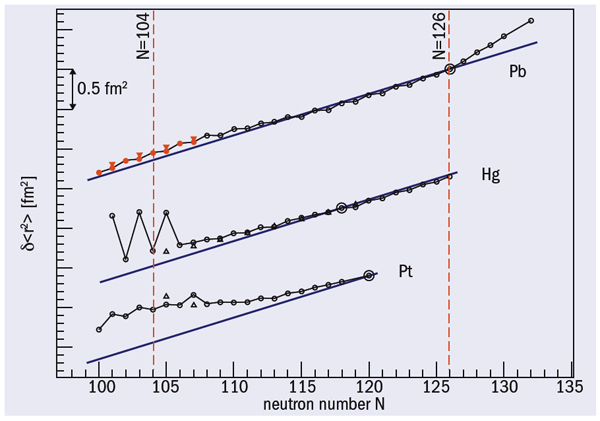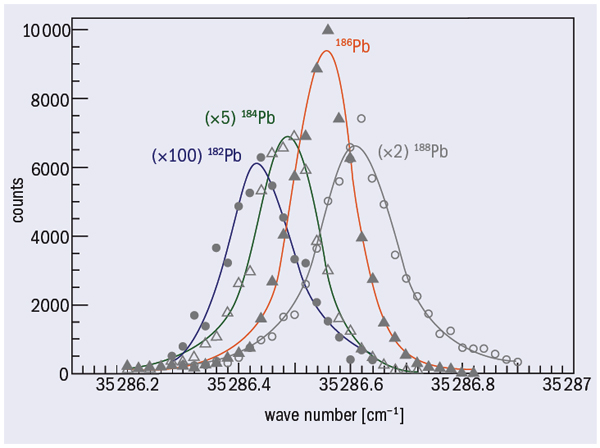# Exotic lead nuclei get into shape at ISOLDE

19 September 2007

Lead nuclei with too few neutrons turn out to be mainly spherical.Fig 1. Changes of mean-square charge radii for lead, mercury, and platinum isotopes. Circles show the ground states and triangles the isomeric states, the latest data are red. Solid lines indicate predictions of the spherical droplet model. (Changes are relative to a reference isotope circled for each element.)

In nature, relatively few nuclei have a spherical shape in their ground state. Examples are 16O, 40Ca, 48Ca and 208Pb, which are “doubly magic”, with numbers of both protons and neutrons corresponding to closed shells in the nuclear shell model. By moving away from the closed shells and increasing the number of valence nucleons, both protons and neutrons, these nuclei can eventually acquire a permanent deformation in their ground state. Experiments reveal that sometimes – due to the complex interplay of single-particle and collective degrees of freedom – both a spherical and deformed shape occur in the same nucleus at low excitation energies. In the region around lead, for example, physicists in the 1970s first observed this “shape co-existence”, using optical spectroscopy at the ISOLDE facility at CERN (Bonn et al. 1972 and Dabkiewicz et al. 1979). Since then, an extensive amount of data has been collected throughout the chart of nuclei (Wood et al. 1992 and Julin et al. 2001).

Some of the best-known examples of shape co-existence are found in neutron-deficient lead nuclei (atomic number or number of protons, Z = 82). The uniqueness of this region is mainly due to three effects. First, the energy gap of 3.9 MeV above the Z = 82 closed proton shell forces the nuclei to adopt a spherical shape in their ground state. However, the energy difference is small enough for a second effect to occur: the creation of “extra” valence proton particles and holes as a result of proton-pair excitation across the gap. Third, a very large neutron valence space between the shell closures with the number of neutrons N = 82 and 126 results in a large number of possible valence neutrons as nuclei approach the neutron mid-shell at N = 104. The strong deformation -driving interaction between the “extra” valence protons and the valence neutrons produces unusually low-lying, deformed oblate (disc-like) and prolate (cigar-like) states in the vicinity of N = 104, where the number of valence neutrons is maximal (Wood et al. 1992). In some cases, the deformation-driving effect is so strong that the deformed state becomes the ground state, as happens near N = 104 in the light isotopes of mercury (Z = 80) and platinum (Z = 78).

Atomic spectroscopy provides direct and model-independent information on the properties of nuclear ground and isomeric states via a determination of hyperfine structure and the isotope shift. These are small effects on atomic energy levels due to the nuclear moments, masses, sizes and shapes of nuclear isotopes, allowing the spins, moments and changes in charge-radii of nuclei to be deduced. In particular, the changes in charge radii determined from the isotope shifts by optical spectroscopy in long isotopic chains have revealed collective nuclear properties clearly.

Figure 1 shows changes of mean-square charge radii (δ<r2>) of lead, mercury and platinum isotopes as a function of the number of neutrons. All the data for the nuclides furthest from stability were determined at ISOLDE by a variety of techniques (Otten 1989 and Kluge and Nörtershäuser 2003). In the 1970s, nuclear-radiation detected optical pumping and laser fluorescence spectroscopy were used, collinear spectroscopy in the 1980s and resonance ionization mass spectroscopy from the late 1980s onwards. Now laser spectroscopy in the laser ion source is used, as described below.

Figure 1 shows how the measured δ<r2> for platinum isotopes develop a distinct deviation from the smoothly decreasing trend expected from the spherical-droplet model. For mercury, a sudden and dramatic change in δ<r2> known as “shape staggering”, occurs between 187Hg and 185Hg (N = 107 and 105 respectively). A similar change occurs between the isomeric (I = 13/2) and ground (I = 1/2) states in 185Hg, in this case, “shape isomerism” or “shape co-existence” (Bonn et al. 1972 and Dabkiewicz et al. 1979). These effects are interpreted as a change from weakly deformed oblate to strongly deformed prolate shapes. The  neutron-deficient lead isotopes are a particularly interesting example of shape co-existence. Theoretical calculations have long suggested the co-existence in these nuclei of three different shapes: spherical, prolate and oblate – hence triple co-existence. Recent particle (α, β) and in-beam studies have found strong evidence for this phenomenon in some of the isotopes from 182Pb to 208Pb.

One of the most spectacular examples is the mid-shell nucleus 186Pb, as indicated in figure 2. Here, studies of the α-decay of the parent nucleus 190Po have revealed a triplet of low-lying (E* < 650 keV)>+ states (Andreyev et al. 2000). These were assigned to co-existing spherical, oblate and prolate shapes, with the spherical state being the ground state. Subsequent in-beam studies identified excited bands built on top of these states. An important question arises, however, concerning the degree of mixing between different configurations. As the excited 0+ states decrease their energy when approaching N = 104 (186Pb), their mixing with the 0+ ground state could increase substantially, an effect that could possibly be seen in the value of the charge radii.

Therefore, the aim of experiment IS483 at ISOLDE was to measure for the first time the isotope shifts in the atomic spectra of the very neutron-deficient nuclei in the region 182Pb to 190Pb, deducing the mean-square charge radii in order to probe the ground state directly (De Witte et al. 2007 and Anderyev et al. 2002). However, the expected production rates were far too low (e.g. 1 ion/s for 182Pb) for the laser spectroscopy  techniques used previously at ISOLDE. Instead, an extremely sensitive spectroscopic technique was employed: resonance ionization spectroscopy in the ion source, first developed at the Petersburg Nuclear Physics Institute in Gatchina for the investigation of rare-earth isotopes (Alkhazov et al. 1992).

The radioactive lead isotopes are produced at ISOLDE in a proton-induced spallation reaction, using protons at 1.4 GeV on a thick (50 g/cm2) target of uranium carbide (UCx). The reaction products diffuse out of the target toward the ionizer tube, which is heated to around 2050 °C. In the tube, a three-step laser ionization process selectively ionizes the lead isotopes. To determine the isotope shift of the appropriate optical spectral line, the laser for the first excitation step is set to a narrow linewidth of 1.2 GHz and its frequency is scanned over the resonance. After ionization and extraction, the radioactive ions are accelerated to 60 keV, mass separated and subsequently implanted in a carbon foil mounted on a rotating wheel at the focal plane of ISOLDE. A circular silicon detector (150 mm2 × 300 μm) placed behind the foil measures the α-radiation during a fixed implantation time, after which the laser frequency is changed and the implantation-measurement cycle repeated again. The implanted lead ions are counted via their characteristic α-decay.Fig. 3 Alpha intensities versus wave number of the laser light used for the first excitation step for the even–even lead isotopes 182–188Pb (De Witte et al. 2007).

Figure 3 shows the intensity of the α-lines as a function of laser frequency for a sequence of nuclei (with even N) from 188Pb to 182Pb. This reveals the optical isotope shift, which allows us to deduce the values of δ<r2> shown in figure 1. Similarly, the experiment also measured isotopes with an odd number of neutrons, 183,185,187Pb, all of them produced in the ground and isomeric states. Note that the “isomer separation” could be obtained by tuning the laser frequency to some specific values at which only one of the isomers is selectively ionized in the cavity and subsequently extracted and analysed.

Figure 1 compares the deduced values of δ<r2> with the predictions of the spherical-droplet model. The deviation from these predictions increases when moving away from the Z = 82 closed proton shell of lead. The large deviation observed for the ground state of the odd-mass mercury isotopes and the odd- and even-mass platinum isotopes around N = 104 has been interpreted as a result of the onset of strong prolate deformation. In the case of lead, from 190Pb downwards, the δ<r2> data show a distinct deviation from the spherical-droplet model. This suggests modest ground-state deformation, but comparisons of the data with model calculations show that δ<r2> is sensitive to correlations in the ground-state wave functions and that the lead isotopes essentially stay spherical in their ground state at – and even beyond – the N = 104 mid-shell region.

This experiment has shown that the extreme sensitivity of the combined in-source laser spectroscopy and α-detection allows us to explore the heavy-mass regions far from stability with isotopes produced at a rate of only a few ions a second (182Pb). An important development would be: to use the isomer shift in the case of odd-mass-number isotopes to ionize nuclei selectively in their ground or isomeric state; to post-accelerate these with the REX-ISOLDE facility; and use the isomerically pure beams of the 13/2+ and 3/2 isomers to investigate, for example, the influence of different spin states of the same incident particle on the reaction mechanism.